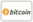Bookcover of On a Class of 3SUM-HARD problems in Computational Geometry
Booktitle:

# On a Class of 3SUM-HARD problems in Computational Geometry

## Cutting a polygon with a line

VDM Verlag Dr. Müller (2009-05-20 )eligible for voucher
ISBN-13:

### 978-3-639-15837-3

ISBN-10:
3639158377
EAN:
9783639158373
Book language:
English
Blurb/Shorttext:
Given a simple polygon P and an integer K > 1, we want to compute the set of straight lines in the Cartesian plane that cut this polygon into exactly K simple polygons. We call this set of lines a K-separator and call this problem the K-separator problem. We present an algorithm that finds the K-separators of an n-vertex simple polygon, for all K > 0, in O(n2) total time. We prove that the decision problem given an integer K > 2 and an edge of the polygon, is there a line through this edge that cuts the polygon in exactly K pieces?, is 3SUM-HARD. For the special case when K = 2, we show that the decision problem can be solved in O(n log(n)) time. Several other complexity results may be obtained. We suspect that the problem of finding the cell of maximum depth is also 3SUM-hard, and as a corollary the problem of identifying the line that cuts the polygon in the maximum possible number of pieces is also 3SUM-hard.
Publishing house:
VDM Verlag Dr. Müller
Website:
http://www.vdm-verlag.de
By (author) :
Ervin Ruci
Number of pages:
76
Published at:
2009-05-20
Stock:
Available
Category:
Geometry
Price:
49.00 €
Keywords:
computational geometry, 3sum hard, polygon cutting### Categories